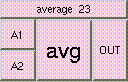AVERAGETHE BOX'S MAIN HELP: This is the average function. The result is the average value of all the inputs. The inputs may be scalars, vectors, or colors, but all inputs must be of the same type. A (vinput): These are the values to be averaged. The inputs may be scalars, vectors, or colors. OUT (output): This is the average function. The result is the average value of all the inputs. The inputs may be scalars, vectors, or colors, but all inputs must be of the same type.

<-- BACK TO Math